# Chapter 1.Introduction

• 发展对监督学习范式的清晰理解，理解术语，并发展一个概念框架来处理未来章节的学习任务
• 学习如何为学习任务的输入编码
• 理解什么是计算图
• 掌握PyTorch的基本知识

## The Supervised Learning Paradigm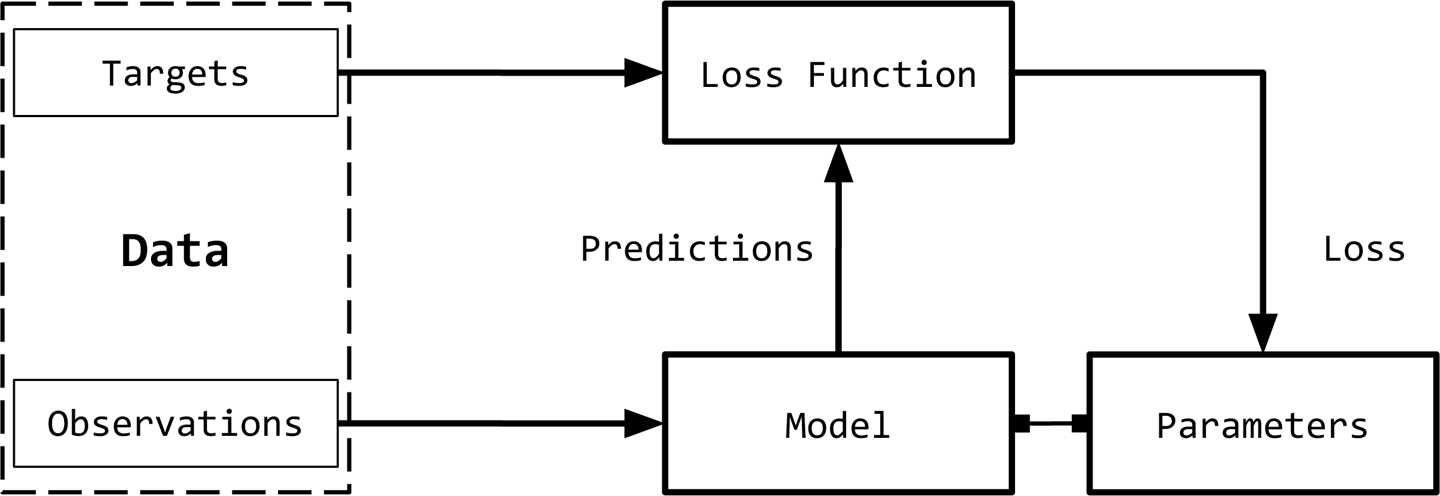Observations：

Targets:

Model:

Parameters:

Predictions:

Loss function:

ŷ =f(X;W)

## Observation and Target Encoding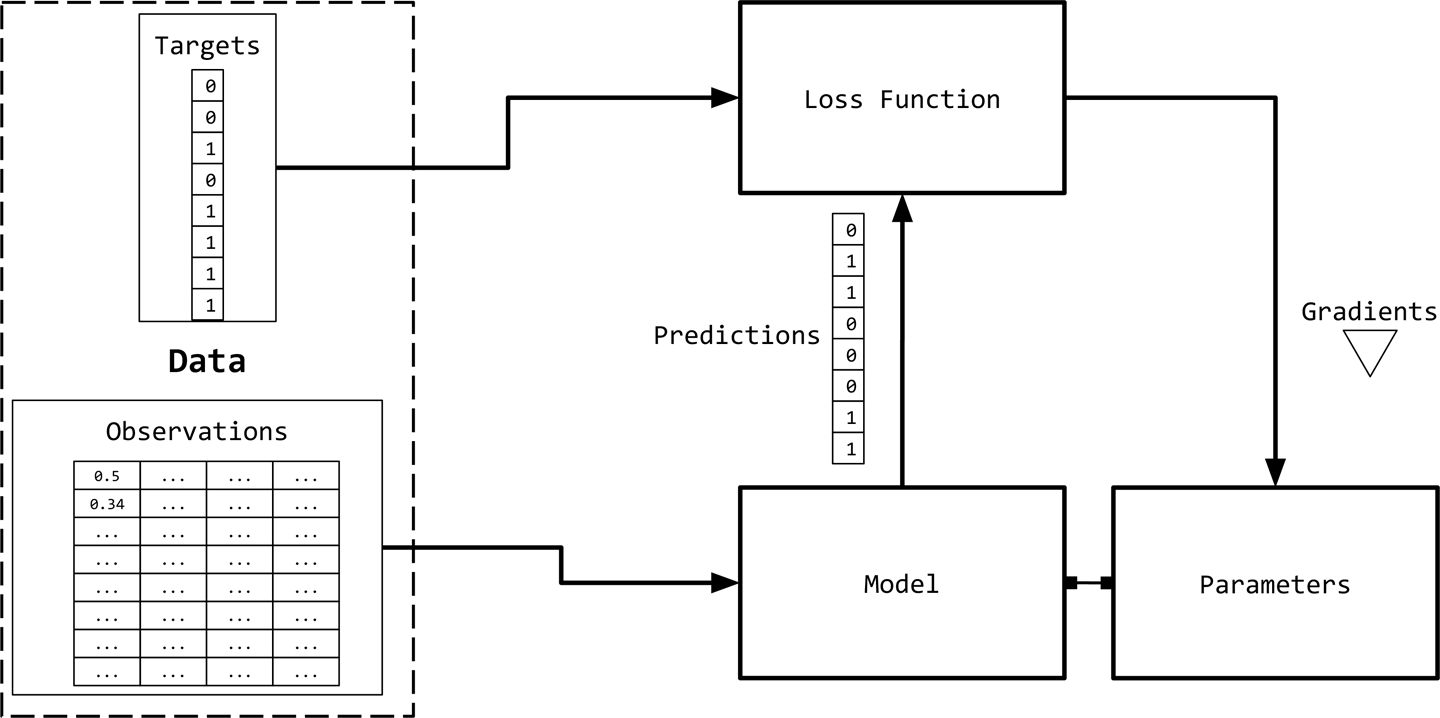### One-Hot Representation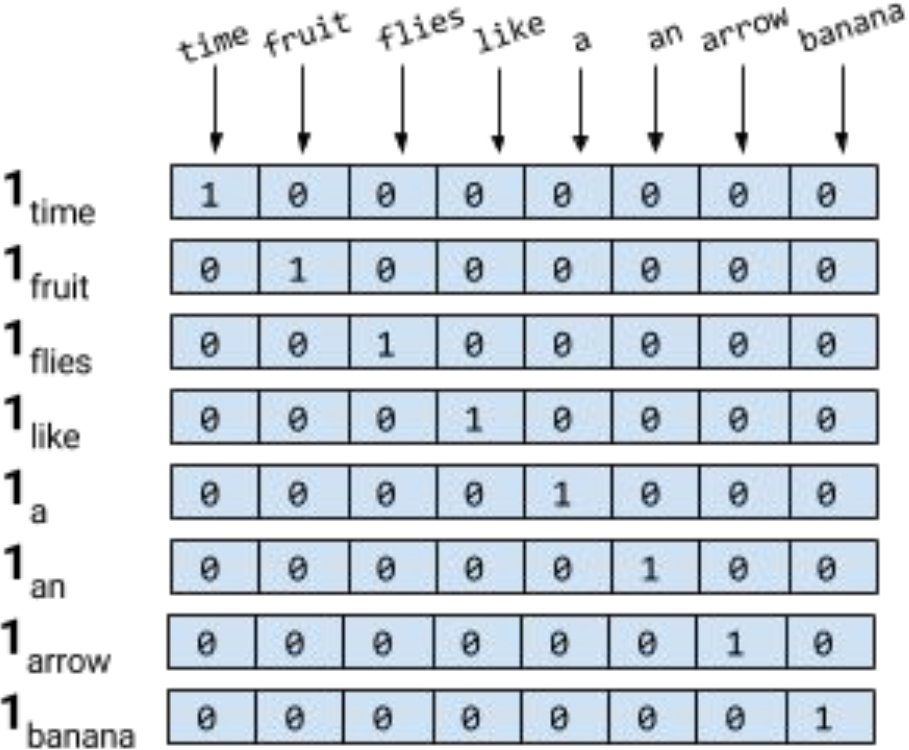NOTE

### TF Representation

Example 1-1. Generating a “collapsed” one-hot or binary representation using scikit-learn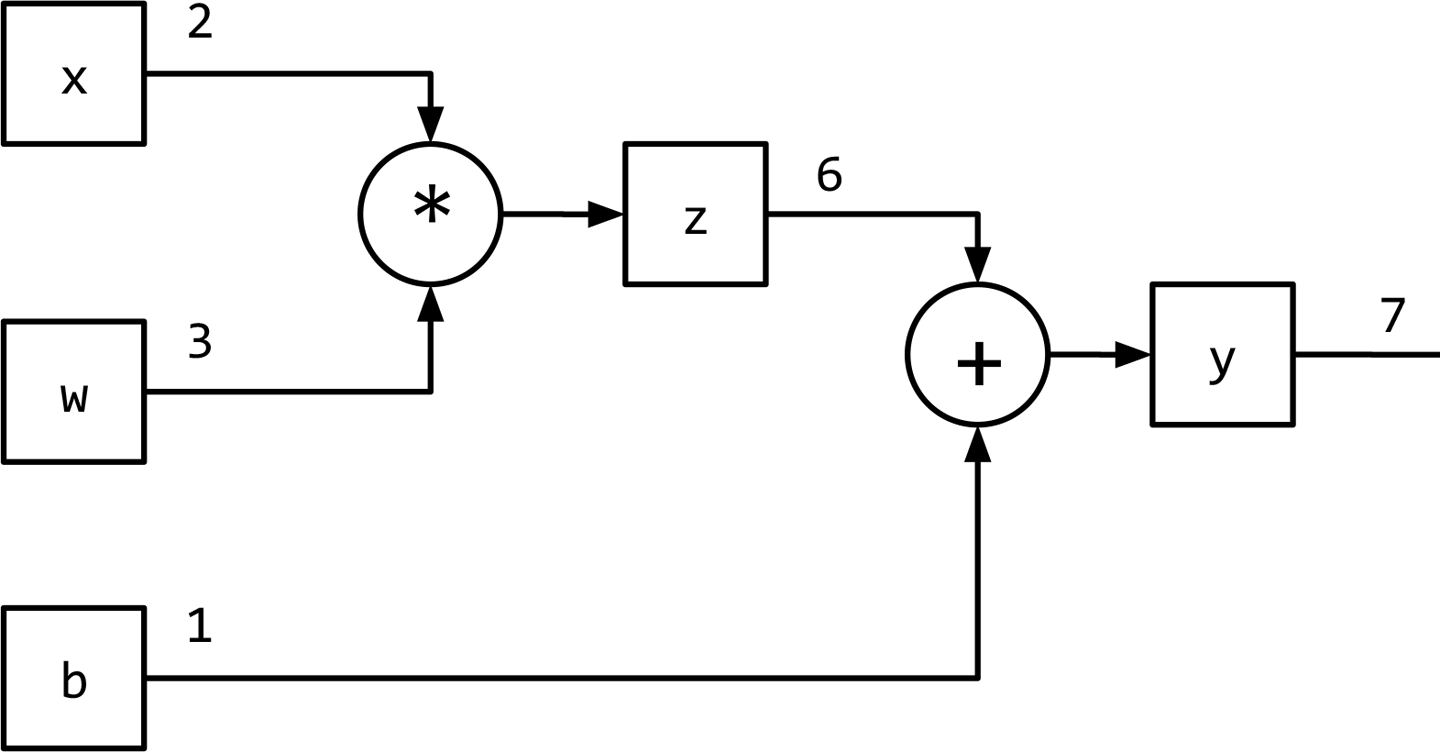### TF-IDF Representation

IDF表示惩罚常见的符号，并奖励向量表示中的罕见符号。 符号w的IDF(w)对语料库的定义为

Example 1-2. Generating TF-IDF representation using scikit-learn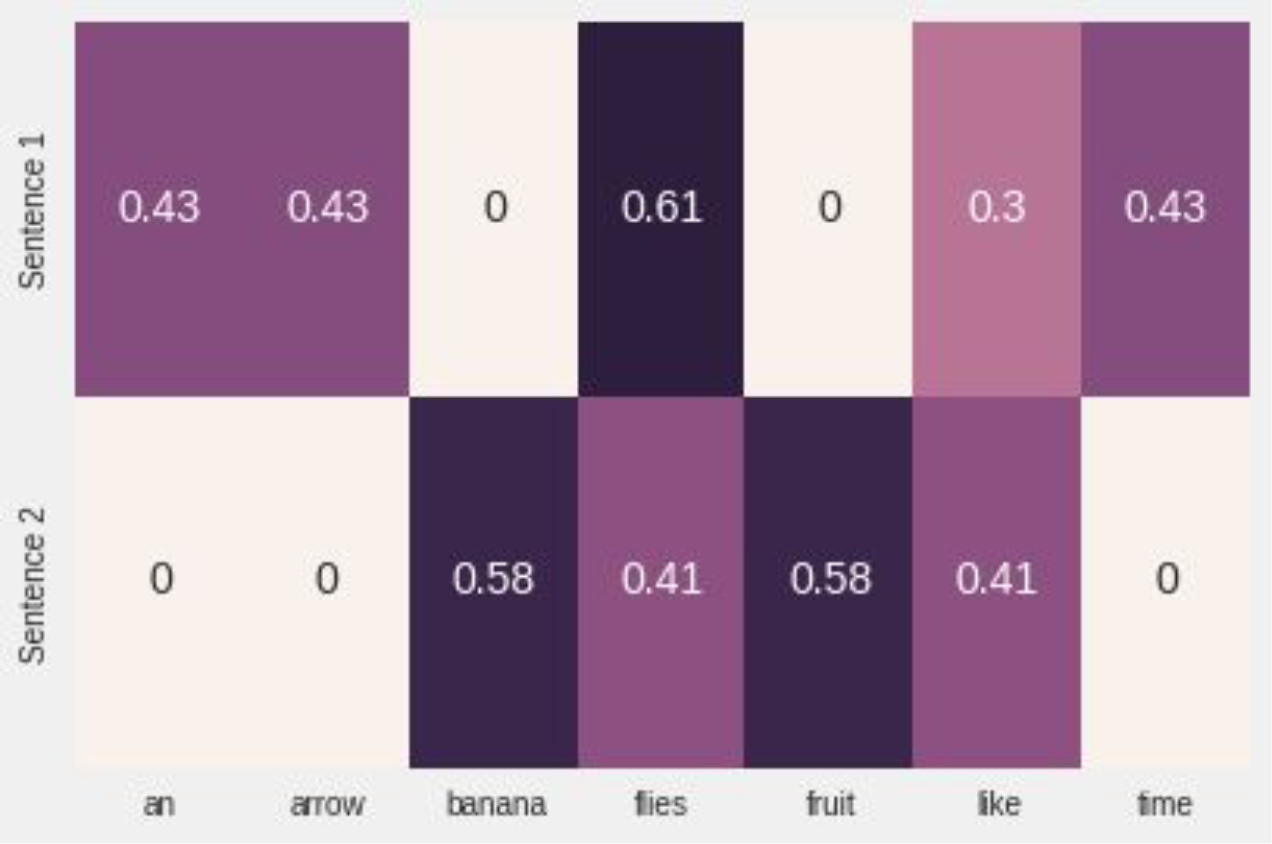## Computational Graphs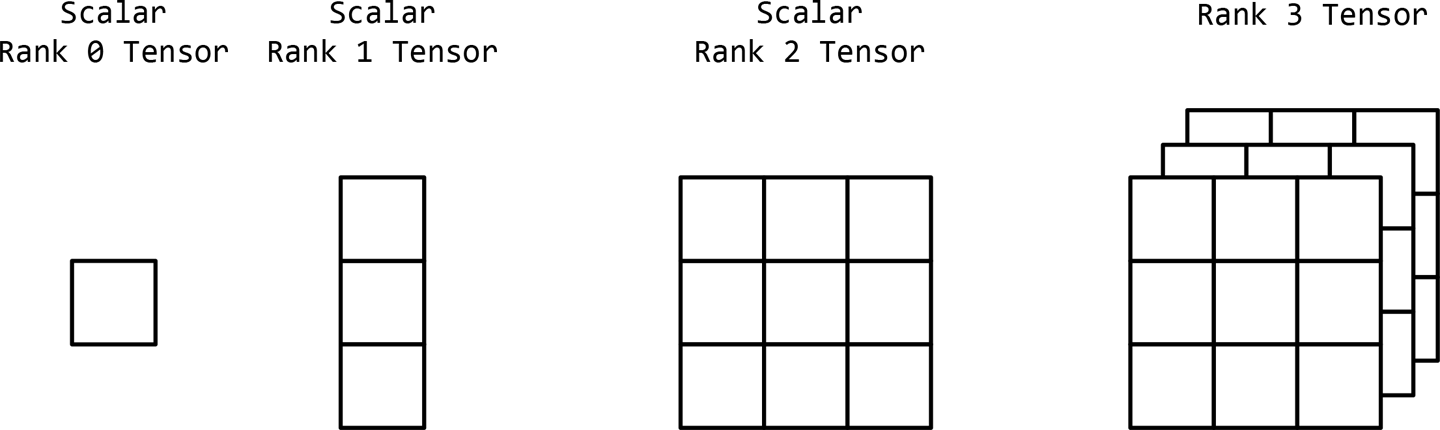## PyTorch Basics

PyTorch是一个优化的张量操作库，它提供了一系列用于深度学习的包。这个库的核心是张量，它是一个包含一些多维数据的数学对象。0阶张量就是一个数字，或者标量。一阶张量(一阶张量)是一个数字数组，或者说是一个向量。类似地，二阶张量是一个向量数组，或者说是一个矩阵。因此，张量可以推广为标量的n维数组，如图1-7所示

• 创建张量
• 操作与张量
• 索引、切片和与张量连接
• 用张量计算梯度
• 使用带有gpu的CUDA张量

NOTE

### Creating Tensors

PyTorch允许我们使用torch包以许多不同的方式创建张量。创建张量的一种方法是通过指定一个随机张量的维数来初始化它，如例1-3所示。

Example 1-3. Creating a tensor in PyTorch with torch.Tensor

Example 1-4. Creating a randomly initialized tensor

Example 1-5. Creating a filled tensor

Example 1-6. Creating and initializing a tensor from lists

Example 1-7. Creating and initializing a tensor from NumPy

### Tensor Types and Size

Example 1-8. Tensor properties

### Tensor Operations

Example 1-9. Tensor operations: addition

Example 1-10. Dimension-based tensor operations

### Indexing, slicing, and joining

Example 1-11. Slicing and indexing a tensor

Example 1-12. Complex indexing: noncontiguous indexing of a tensor

Example 1-13. Concatenating tensors

PyTorch还在张量上实现了高效的线性代数操作，如乘法、逆和trace，如示例1-14所示。

Example 1-14. Linear algebra on tensors: multiplication

### Tensors and Computational Graphs

PyTorch张量类封装了数据(张量本身)和一系列操作，如代数操作、索引操作和整形操作。然而,1-15所示的例子,当requires_grad布尔标志被设置为True的张量,记账操作启用,可以追踪的梯度张量以及梯度函数,这两个需要基于促进梯度学习讨论“监督学习范式”。

Example 1-15. Creating tensors for gradient bookkeeping

### CUDA Tensors

PyTorch使创建这些CUDA张量变得非常容易(示例1-16)，它将张量从CPU传输到GPU，同时维护其底层类型。PyTorch中的首选方法是与设备无关，并编写在GPU或CPU上都能工作的代码。在下面的代码片段中，我们首先使用torch.cuda.is_available()检查GPU是否可用，然后使用torch.device检索设备名。然后，将实例化所有未来的张量，并使用.to(device)方法将其移动到目标设备。

Example 1-16. Creating CUDA tensors

Example 1-17. Mixing CUDA tensors with CPU bound tensors

# Exercises:

.

1. Create a 2D tensor and then add a dimension of size 1 inserted at dimension 0.

2. Remove the extra dimension you just added to the previous tensor.

3. Create a random tensor of shape 5x3 in the interval [3, 7)

4. Create a tensor with values from a normal distribution (mean=0, std=1).

5. Retrieve the indexes of all the nonzero elements in the tensor torch.Tensor([1, 1, 1, 0, 1]).

6. Create a random tensor of size (3,1) and then horizontally stack 4 copies together.

7. Return the batch matrix-matrix product of two 3-dimensional matrices (a=torch.rand(3,4,5), b=torch.rand(3,5,4)).

8. Return the batch matrix-matrix product of a 3D matrix and a 2D matrix (a=torch.rand(3,4,5), b=torch.rand(5,4)).

# Solutions

9. a = torch.rand(3, 3)
a.unsqueeze(0)
10. a.squeeze(0)
11. 3 + torch.rand(5, 3) * (7 - 3)
12. a = torch.rand(3, 3)
a.normal_()
13. a = torch.Tensor([1, 1, 1, 0, 1])
torch.nonzero(a)

14. a = torch.rand(3, 1)
a.expand(3, 4)

15. a = torch.rand(3, 4, 5)
b = torch.rand(3, 5, 4)
torch.bmm(a, b)

16. a = torch.rand(3, 4, 5)
b = torch.rand(5, 4)
torch.bmm(a, b.unsqueeze(0).expand(a.size(0), * b.size()))

# Summary

TF应该很容易理解就是计算词频,IDF衡量词的常见程度.为了计算IDF我们需要事先准备一个语料库用来模拟语言的使用环境,如果一个词越是常见,那么式子中分母越大,逆文档频率越接近0.这里分母+1是为了避免分母为0的情况出现

TF-IDF可以很好的实现提取文章中关键词的目的.

-------------本文结束感谢您的阅读-------------